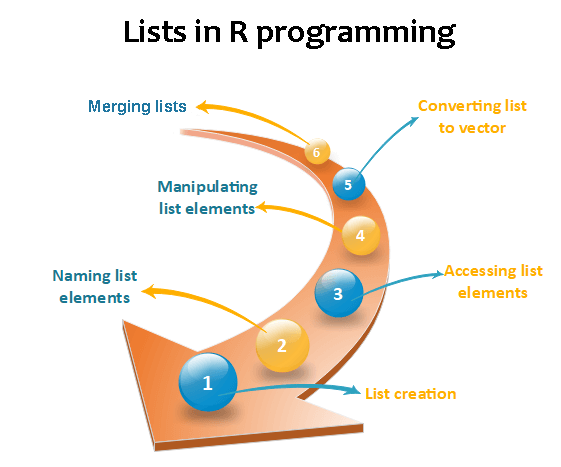# R Lists

In R, lists are the second type of vector. Lists are the objects of R which contain elements of different types such as number, vectors, string and another list inside it. It can also contain a function or a matrix as its elements. A list is a data structure which has components of mixed data types. We can say, a list is a generic vector which contains other objects.

### Example

vec <- c(3,4,5,6)
char_vec<-c(“shubham”,“nishka”,“gunjan”,“sumit”)
logic_vec<-c(TRUE,FALSE,FALSE,TRUE)
out_list<-list(vec,char_vec,logic_vec)
out_list

Output:

``````[]
 3 4 5 6
[]
 "shubham" "nishka"  "gunjan"  "sumit"
[]
  TRUE FALSE FALSE  TRUE
``````## Lists creation

The process of creating a list is the same as a vector. In R, the vector is created with the help of c() function. Like c() function, there is another function, i.e., list() which is used to create a list in R. A list avoid the drawback of the vector which is data type. We can add the elements in the list of different data types.

Syntax

list()

Example 1: Creating list with same data type

list_1<-list(1,2,3)
list_2<-list(“Shubham”,“Arpita”,“Vaishali”)
list_3<-list(c(1,2,3))
list_4<-list(TRUE,FALSE,TRUE)
list_1
list_2
list_3
list_4

Output:

``````[]
 1
[]
 2
[]
 3

[]
 "Shubham"
[]
 "Arpita"
[]
 "Vaishali"

[]
 1 2 3

[]
 TRUE
[]
 FALSE
[]
 TRUE
``````

Example 2: Creating the list with different data type

list_data<-list(“Shubham”,“Arpita”,c(1,2,3,4,5),TRUE,FALSE,22.5,12L)
print(list_data)

In the above example, the list function will create a list with character, logical, numeric, and vector element. It will give the following output

Output:

``````[]
 "Shubham"
[]
 "Arpita"
[]
 1 2 3 4 5
[]
 TRUE
[]
 FALSE
[]
 22.5
[]
 12
``````

## Giving a name to list elements

R provides a very easy way for accessing elements, i.e., by giving the name to each element of a list. By assigning names to the elements, we can access the element easily. There are only three steps to print the list data corresponding to the name:

1. Creating a list.
2. Assign a name to the list elements with the help of names() function.
3. Print the list data.

Let see an example to understand how we can give the names to the list elements.

Example

``````# Creating a list containing a vector, a matrix and a list.
list_data <- list(c("Shubham","Nishka","Gunjan"), matrix(c(40,80,60,70,90,80), nrow = 2),
list("BCA","MCA","B.tech"))

# Giving names to the elements in the list.
names(list_data) <- c("Students", "Marks", "Course")

# Show the list.
print(list_data)
``````

Output:

``````\$Students
 "Shubham" "Nishka"  "Gunjan"

\$Marks
[,1] [,2] [,3]
[1,]   40   60   90
[2,]   80   70   80

\$Course
\$Course[]
 "BCA"

\$Course[]
 "MCA"

\$Course[]
 "B. tech."``````